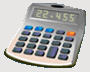DosageHelp.com Helping Nursing Students Prepare for Medication Exams By Explaining Dosage Calculations

# Amount in IV Fluid Questions

Given a volume of IV fluid and a dosage expressed in percent, what is the mass of a particular dosage?

Formula:
 Concentration % 100
x Volume (mL)  =  Y (Dosage Amount in g)

Example: Calculate the amount of dextrose in 1000 mL D5W.
 Concentration % 100
x Volume (mL)  =  Y (Dosage Amount in g)
 5% 100
x 1000 mL  =  50 g

Example: Calculate the amount of sodium chloride in 2000 mL NS.
Recall NS is 0.9% NaCl (sodium chloride)
 Concentration % 100
x Volume (mL)  =  Y (Dosage Amount in g)
 0.9% 100
x 2000 mL  =  18 g

Home Dosage Question Steps Unit Conversion Mass for Mass Mass/Liquid For Liquid IV Terms & Abbreviations Amount in IV Fluid Volume/Time - IV mL Rate Volume/Time - IV Drop Rate Fluid Maintenance Dosage By Weight Mass/Time - IV mL Rate Practice Questions Question of the Day Tell a Friend Print a Flyer